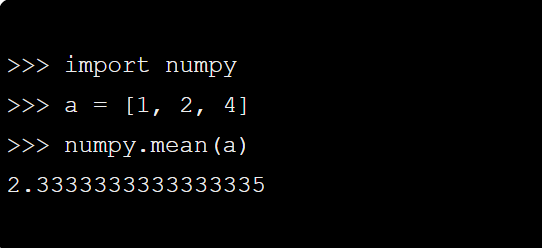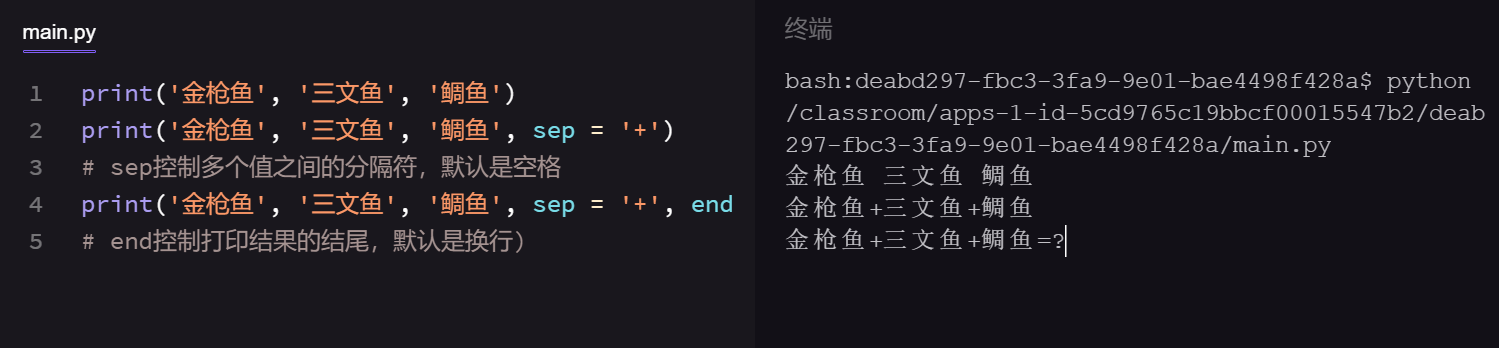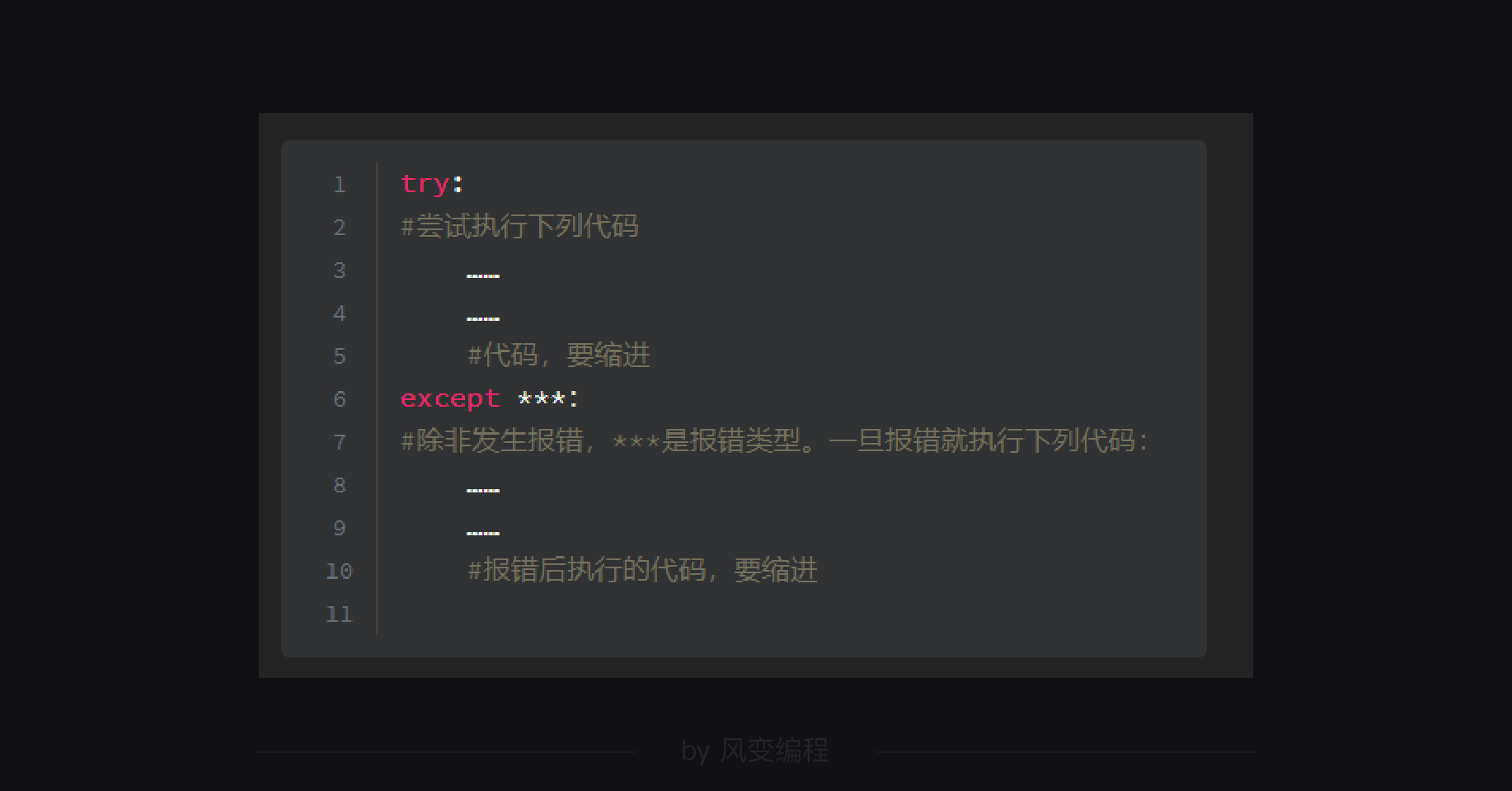## (十）用numpy库求平均值，表示数组

NumPy有一个numpy.mean，它是一个算术平均值，import numpy as np  # 导入 numpy库，下面出现的 np 即 numpy库

scores1 =  [91, 95, 97, 99, 92, 93, 96, 98]
scores2 = []

average = np.mean(scores1)  # 一行解决。
print('平均成绩是：{}'.format(average))

for score in scores1:
if score < average:
scores2.append(score) # 少于平均分的成绩放到新建的空列表中
print(' 低于平均成绩的有：{}'.format(scores2))  # 上个关卡选做题的知识。

# 下面展示一种NumPy数组的操作，感兴趣的同学可以自行去学习哈。
scores3 = np.array(scores1)
print(' 低于平均成绩的有：{}'.format(scores3[scores3<average]))## （十一）print函数相关

print函数的完整参数为：

print(*objects, sep = ' ', end = '\n', file = sys.stdout, flush = False)

print('金枪鱼', '三文鱼', '鲷鱼')
print('金枪鱼', '三文鱼', '鲷鱼', sep = '+')
# sep控制多个值之间的分隔符，默认是空格
print('金枪鱼', '三文鱼', '鲷鱼', sep = '+', end = '=?')
# end控制打印结果的结尾，默认是换行)## （十二）取整

1.向上取整：ceil函数

import math

# 人力计算
def estimated_number(size,time):
number = math.ceil(size * 80 / time)
print('项目大小为%.1f个标准项目，如果需要在%.1f个工时完成，则需要人力数量为：%d人' %(size,time,number))

# 调用人力计算函数
estimated_number(1,60)2.四舍五入：round()

round(4.4)
->>4
round(4.6)
->>5

## （十三）index()函数

index() 函数用于找出列表中某个元素第一次出现的索引位置。

num = [0,1,0,1,2]
print(num.index(1))  # 数字1首先出现的索引位置是list（索引位置从0开始）。
print(num.index(2))  # 数字2首先出现的索引位置是list。

1
4

import random

# 出拳
punches = ['石头','剪刀','布']
computer_choice = random.choice(punches)
user_choice = ''
user_choice = input('请出拳：（石头、剪刀、布）')  # 请用户输入选择
while user_choice not in punches:  # 当用户输入错误，提示错误，重新输入
print('输入有误，请重新出拳')
user_choice = input()

# 亮拳
print('————战斗过程————')
print('电脑出了：%s' % computer_choice)
print('你出了：%s' % user_choice)

# 胜负
print('—————结果—————')
if user_choice == computer_choice:  # 使用if进行条件判断
print('平局！')
# 电脑的选择有3种，索引位置分别是：0石头、1剪刀、2布。
# 假设在电脑索引位置上减1，对应：-1布，0石头，1剪刀，皆胜。
elif user_choice == punches[punches.index(computer_choice)-1]:
print('你赢了！')
else:
print('你输了！')

## （十四）try...except语句print('\n欢迎使用除法计算器！\n')

while True:
try:
x = input('请你输入被除数：')
y = input('请你输入除数：')
z = float(x)/float(y)
print(x,'/',y,'=',z)
break  # 默认每次只计算一次，所以在这里写了 break。
except ZeroDivisionError:  # 当除数为0时，跳出提示，重新输入。
print('0是不能做除数的！')
except ValueError:  # 当除数或被除数中有一个无法转换成浮点数时，跳出提示，重新输入。
print('除数和被除数都应该是整值或浮点数！')

# 方式2：将两个（或多个）异常放在一起，只要触发其中一个，就执行所包含的代码。
# except(ZeroDivisionError,ValueError):
#     print('你的输入有误，请重新输入！')

# 方式3：常规错误的基类，假设不想提供很精细的提示，可以用这个语句响应常规错误。
# except Exception:
#     print('你的输入有误，请重新输入！')


## （十五）os模块实现写入文件

import os

list_test = ['一弦一柱思华年。\n','只是当时已惘然。\n']

with open ('poem3.txt','r') as f:
# 语法：os.replace(file1,file2)，将file1重命名为file2，将其替代。